﻿ Input-state Linearization of Mechanical SystemPublications are Open
Access in this journal
Article Versions
Export Article
• Normal Style
• MLA Style
• APA Style
• Chicago Style
Research Article
Open Access Peer-reviewed

Input-state Linearization of Mechanical System

Tomáš Lipták , Michal Kelemen, Alexander Gmiterko, Ivan Virgala, Darina Hroncová
American Journal of Mechanical Engineering. 2017, 5(6), 298-302. DOI: 10.12691/ajme-5-6-13
Published online: December 15, 2017

Abstract

The article deals with the issue of input-state linearization of the mechanical systems. The introductory part of article contains theory about exact linearization that we used. Further we explained the basic principle and procedure of input-state linearization. Then it contains the determination of state space for mechanical systems, the computing of vector fields, the investigation of controllability and involutivity of the systems and the calculation of input and state transformation.

1. Introduction

The control theory of nonlinear systems has been receiving increasing attention in recent years, both for its technical importance as well as for its impact in various fields of application. In strategic areas, such as aerospace, chemical and petrochemical industries, bioengineering and robotics, a new practical application for this tool appears every day 1.

The actual physical systems exhibit the features of nonlinearity and additionally have time-varying parameters. The study of such systems boils down to the analysis of mathematical model which in general case is nonlinear. In most cases, there is no analytical solution of the problem (which is frequently sought). As shown in 2, 3, 4, 5, although they are universal and applicable to any number of differential equations, they generate a numerical solution (compiled in the form of tables, graphs, etc.). Therefore, in the search for analytical solutions the transformation of nonlinear description into a linear one by linearization (ensuring the equilibrium of the system dynamics) is very useful in solving practical problems.

The modern theory of nonlinear systems, in particular the geometric approach, has acquired major significance in the linearization of nonlinear systems. The application of geometric approach to solve nonlinear problems initiated by Brockett in 5 was used in control theory with observability and controllability of the systems 6, 7 taken into account. The methods of differential geometry allowed the development of efficient techniques for the analysis and synthesis of such systems.

System nonlinearity is characterized when at least one component or subsystem is nonlinear. The classic methods used in the study of nonlinear systems are not usually applied to the nonlinear systems. It is necessary to use other methods to study the control of these systems. When the nonlinear effects of systems become significant, nonlinear control techniques generally fail to produce the desired performance. In these cases, there are several nonlinear control techniques that have proven to supply better results.

The exact or feedback linearization consists of full or partial compensation of system nonlinearities by manner that the final system should have less number of nonlinearities or the final system should behave as linear between input and output. The compensation of nonlinearities can be done globally in whole state space or locally in some area. Methods of exact linearization can be divided to two groups: input-state linearization and input-output linearization 8, 9.

In this article we focused on input-state linearization of nonlinear the mechanical system with one degree of freedom and one-link manipulator.

2. Input-state Linearization

We deal with input-state linearization for nonlinear affine system with one input (without output) represented by state equation: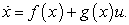(1)

This system is known as linear in the control and linear in the input, among other denominations. The functions f(x) and g(x) are C vectors with field in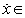Rn. The system in form (1) is input-state linearizable if there is a region Ω in Rn that meets the following conditions:

1) the vector fields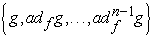are linearly independents in Ω,

2) the set of vectors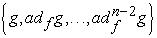are involutive.

The first condition indicates the system controllability in which the vector fieldsare equivalent to the controllability matrix for linear systems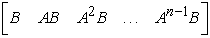.

The second involutivity condition enables to find out a new vector of linear state through the states feedback. 10

2.1. The Procedure of Input-state Linearization

If the aforementioned conditions were satisfied, then it is possible to continue by this manner 11:

1) for given system (1) we compute vector fields,

2) we investigate condition of controllability and involutivity,

3) if previous conditions are satisfied, we find the first state T1 from equations: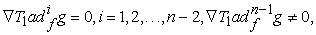(2)

4) we compute the state transformation: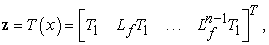(3)

and input transformation: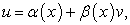(4)

where: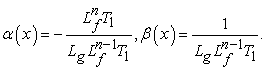(5)
• Figure 1. The scheme of input-state linearization

The scheme of input-state linearization we ca see in the previous figure Figure 1.

3. The Mechanical System with One Degree of Freedom

We will show input-state linearization on example of the mechanical system with one degree of freedom - the second order system (Figure 2). The mechanical system is created by connecting the rigid body with the frame through the spring and damper. This system is described one equation: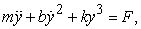(6)

where m is the mass of rigid body, b is the constant of nonlinear viscous damping, k is the constant of spring and F is input force acting to rigid body.

• Figure 2. The mechanical system with one degree of freedom
3.1. The State-space Form

At first we must create the state space from motion equation (6). We choose the state vector in the form: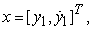(7)

and the state-space form is: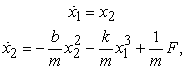(8)

or in matrix form: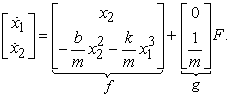(9)
3.2. The Computing of Vector Fields

The dynamic system is the second order, thus we have two state equations and therefore we have to obtain two independent vector fields according to relation 12: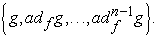(10)

The first vector field has the form: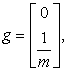(11)

the second vector fields we determine using Lie bracket: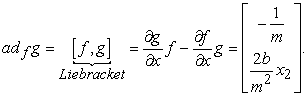(12)
3.3. The Investigation of Controllability and Involutivity of the System

Now we can create controllability matrix using computed vector fields 13: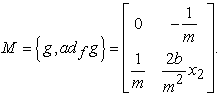(13)

The system is controllable if the rank of controllability matrix is equal to order of system or determinant of this matrix is nonzero. The rank of matrix is h(M) = 2 and it is equal to order of system and also the determinant is nonzero, i.e.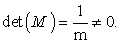Thus individual vector fields are linearly independent and therefore the system is controllable.

At the investigation of involutivity is needed to do the new combination of Lie bracket from existing vector fields: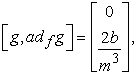(14)

and now we can compare original vector field (13) and expanded vector field 14: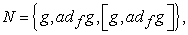(15)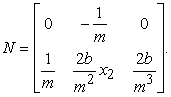(16)

The rank of original and expanded vector field is the same, thus h(M) = h(N) = 2, i.e. the system is involutive.

The system is controllable and involutive, which satisfy the conditions to apply input-state linearization.

3.4. The Finding of the First State T1

In this chapter we will choose suitable the first state T1 according to relation (2):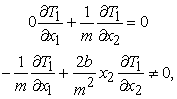(17)

this results in that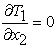and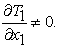In order to satisfy the last equation,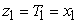was chosen. 15

3.5. The Calculation of State and Input Transformation

Then new state variables according to relation (3) are: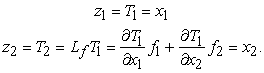(18)

For input transformation we use relations (4) and (5):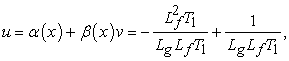(19)

where: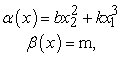(20)

and then input is: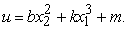(21)

Now we can substitute individual previous relations to the scheme of input-state linearization (Figure 2).

In this chapter we will show input-state linearization on example of one-link manipulator - the fourth order system (Figure 3). The mechanical system is created by connecting output shaft with the load through torsion spring to shaft of drive. This system is described by two equations: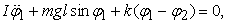(22)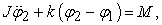(23)

where J is the moment of inertia of the drive, I is the moment of inertia of the manipulator, l is the distance of the output shaft to the center of gravity of the manipulator, m is the mass of the manipulator, g is the gravity acceleration, k is the torsion constant of the spring, ϕ1 is the angle of rotation of the manipulator, ϕ2 is the angle of rotation of the motor shaft and M is the drive torque.

• Figure 3. The one-link manipulator
4.1. The State-space Form

At first we must create the state space from motion equations (22) and (23). We have the state vector in the form: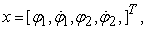(24)

and the state-space form is: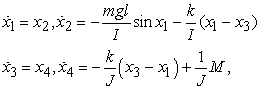(25)

or in matrix form: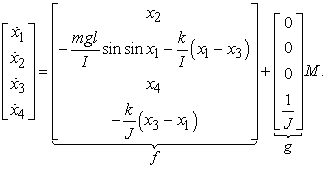(26)
4.2. The Computing of Vector Fields

The dynamic system is the fourth order thus we have four state equations and therefore we have to obtain four independent vector fields according to relation 12:(27)

The first vector field has the form: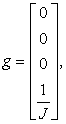(28)

the second vector fields we determine using Lie bracket: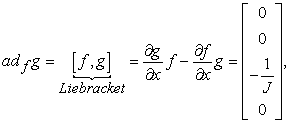(29)

the third vector field: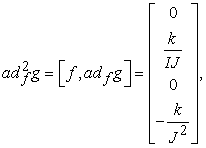(30)

and the last fourth vector field: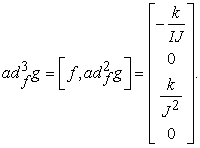(31)
4.3. The Investigation of Controllability and Involutivity of the System

Now we can create controllability matrix using computed vector fields 13: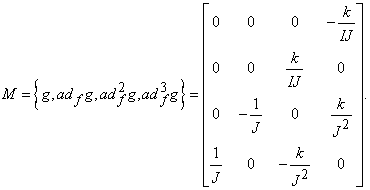(32)

The rank of matrix is h(M) = 4 and it is equal to order of system and also the determinant is nonzero, i.e.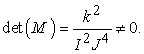Thus individual vector fields are linearly independent and therefore the system is controllable.

At the investigation of involutivity is needed to do the new combination of Lie bracket from existing vector fields: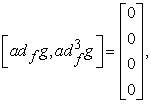(33)

and now we can compare original vector field (32) and expanded vector field 14: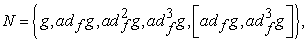(34)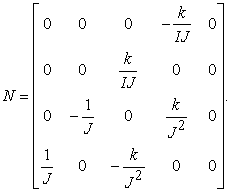(35)

The rank of original and expanded vector field is the same, thus h(M) = h(N) 4, i.e. the system is involutive.

The system is controllable and involutive, which satisfy the conditions to apply input-state linearization.

4.4. The Finding of the First State T1

In this chapter we will choose suitable the first state T1 according to relation (2):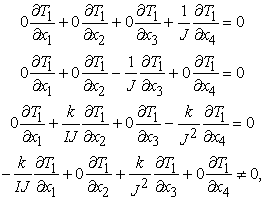(36)

this results in that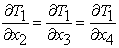andIn order to satisfy the last equation,was chosen 15.

4.5. The Calculation of State and Input Transformation

Then new state variables according to relation (3) are: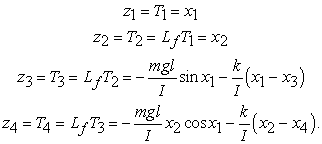(37)

For input transformation we use relations (4) and (5):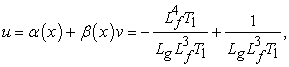(38)

where: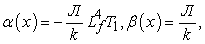(39)

and then input is: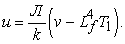(40)

At linearized systems we can already design the regulators using known methods of linear synthesis. In the case mechanical system with one degree we have to design the regulator for two state variables and in the case the one-link manipulator the regulator for four state variables.

5. Conclusion

The aim of paper was to introduce the use of exact linearization at linearizing of mechanical systems. At first we discussed about theory of exact linearization- input-state linearization. This linearization has some steps that is needed to do. After computing of vector fields must be done the investigation of controllability and involutivity condition. If these conditions are satisfied we can find the first state and then determine state and input transformation. This type of linearization we presented on the second order system - mechanical system with one degree of freedom and on the fourth order system - one-link manipulator.

Acknowledgements

The work has been accomplished under the research project No. VEGA 1/0872/16 „Research of synthetic and biological inspired locomotion of mechatronic systems in rugged terrain”.

References

  J. J. Slotine and L. Weiping, Applied Nonlinear Control, Prentice-Hall, Englewood Cliffs, NJ, USA, 1991. In article View Article  LAMBERT, J. D.: Numerical Methods for Ordinary Differential Systems, John Wiley & Sons, Chichester, 1991. In article View Article  QUARTERONI, A.-SACCO, R.-SALERI, F.: Numerical Mathematics, Springer-Verlag, New York, 2000. In article View Article  ATKINSON, K.-HAN, W.-STEWART, D.: Numerical Solution of Ordinary Differential Equations, John Wiley & Sons, Inc., Hoboken, New Jersey, 2009. In article  NAJM, F. N.: Circuit Simulation, John Wiley & Sons, Inc., Hoboken, 2010. In article  BROCKETT, R. W.: Nonlinear Systems and Differential Geometry, Proc. Of IEEE 64 No. 1 (1976), 61-71. In article View Article  JAKUBCZYK, B.-RESPONDEK, W.: On Linearization of Control Systems, Bull. Acad. Polonaise, Sci., Ser. Sci. Math. 28 (1980), 517-522. In article View Article  BYRNES, C. I.-ISIDORI, A.: Local Stabilization of Minimum Phase Nonlinear Systems, Syst. Control Lett. 11 (1988), 9-19. In article View Article  J. Bronislaw, “Introduction to geometric nonlinear control; controllability and lie bracket,” in Mathematical Control Theory, Lectures Notes of a Minicourse, pp. 107-168, Abdus Salam International Center Theoret Physics, Trieste, Italy, 2002. In article View Article  A. J. Krener, “Approximate linearization by state feedback and coordinate change,” Systems & Control Letters, vol. 5, no. 3, pp. 181-185, 1984. In article View Article  S. Renou and S. Saydy, “Real time control of an inverted pendulum based on approximate linearization,” in Proceedings of the Canadian Conference on Electrical and Computer Engineering (CCECE ’96), vol. 2, pp. 502-504, May 1996. In article View Article  N. Bedrossian, Nonlinear control using linearizing transformations, Ph.D. thesis, Department of Mechanical Engineering, Massachusetts Institute of Technology, Cambridge, Mass, USA, 1991. In article View Article  N. S. Bedrossian, “Approximate feedback linearization: The cart-pole example,” in Proceedings of the IEEE International Conference on Robotics and Automation, vol. 3, pp. 1987-1992, 1992. In article View Article  J. Deutscher and C. Schmid, “A state space embedding approach to approximate feedback linearization of single input nonlinear control systems,” International Journal of Robust and Nonlinear Control, vol. 16, no. 9, pp. 421-440, 2006. In article View Article  L. Guzzella and A. Isidori, “On approximate linearization of nonlinear control systems,” International Journal of Robust & Nonlinear Control, vol. 3, no. 3, pp. 261-276, 1993. In article View Article

Published with license by Science and Education Publishing, Copyright © 2017 Tomáš Lipták, Michal Kelemen, Alexander Gmiterko, Ivan Virgala and Darina HroncováThis work is licensed under a Creative Commons Attribution 4.0 International License. To view a copy of this license, visit http://creativecommons.org/licenses/by/4.0/